Скачать презентацию Part 1 Thinking about the meaning of Division

2b857fb9490b874734b6961e132f512a.ppt

• Количество слайдов: 29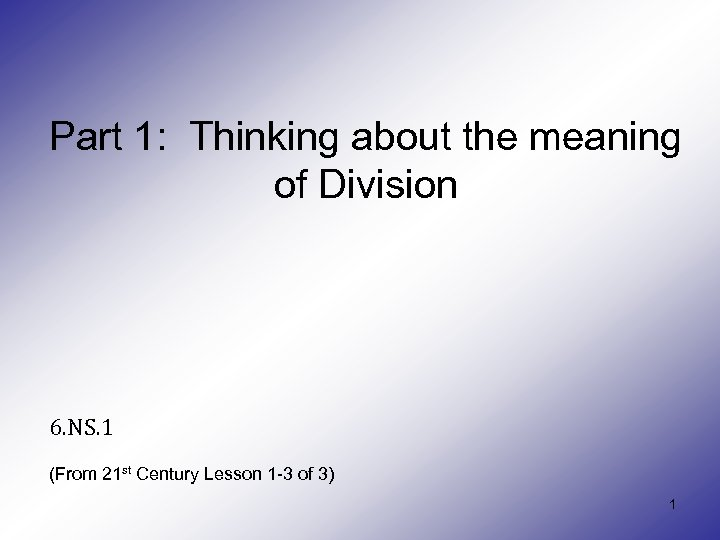Part 1: Thinking about the meaning of Division 6. NS. 1 (From 21 st Century Lesson 1 -3 of 3) 1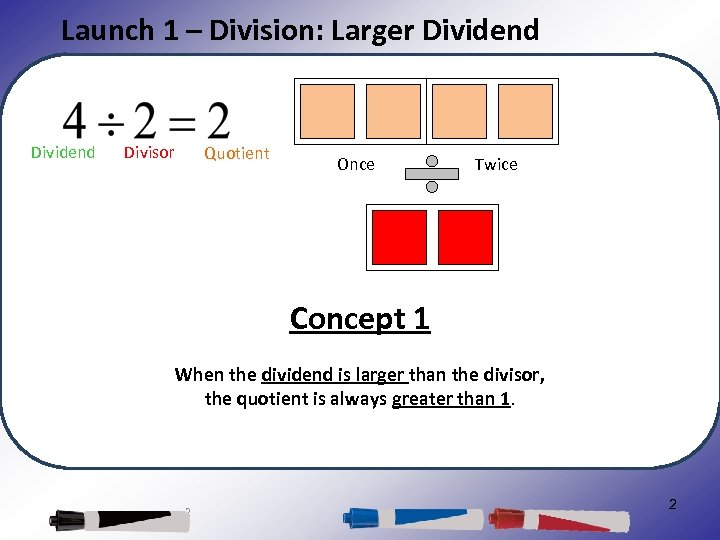Launch 1 – Division: Larger Dividend Divisor Quotient Once Twice Concept 1 When the dividend is larger than the divisor, the quotient is always greater than 1. 2 2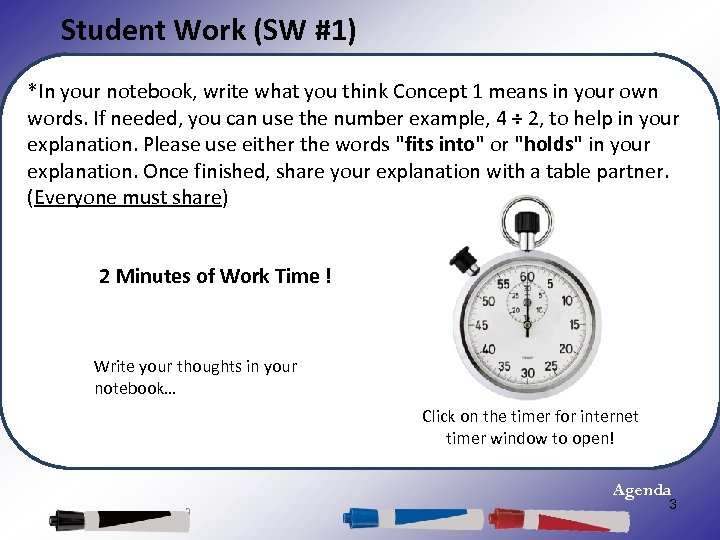Student Work (SW #1) *In your notebook, write what you think Concept 1 means in your own words. If needed, you can use the number example, 4 ÷ 2, to help in your explanation. Please use either the words "fits into" or "holds" in your explanation. Once finished, share your explanation with a table partner. (Everyone must share) 2 Minutes of Work Time ! Write your thoughts in your notebook… Click on the timer for internet timer window to open! Agenda 3 3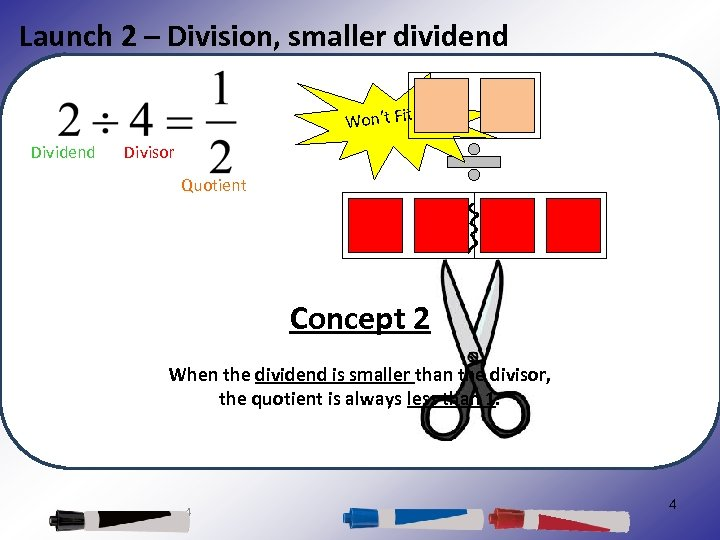Launch 2 – Division, smaller dividend Won’t Fit Dividend Divisor Quotient Concept 2 When the dividend is smaller than the divisor, the quotient is always less than 1. 4 4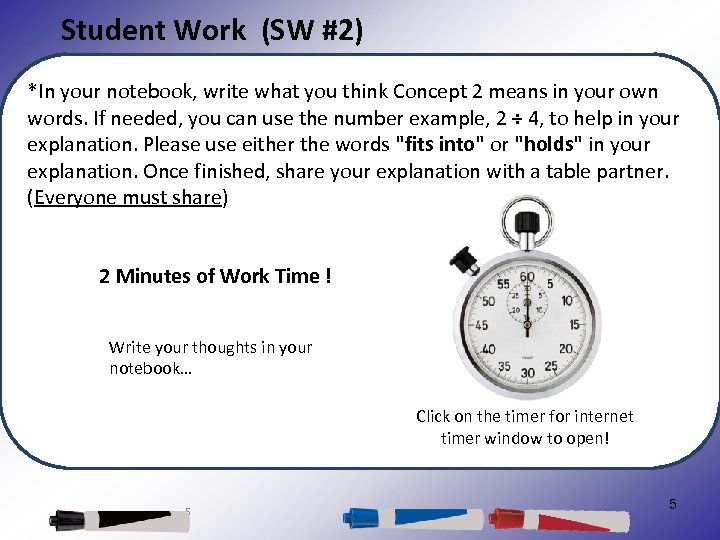Student Work (SW #2) *In your notebook, write what you think Concept 2 means in your own words. If needed, you can use the number example, 2 ÷ 4, to help in your explanation. Please use either the words "fits into" or "holds" in your explanation. Once finished, share your explanation with a table partner. (Everyone must share) 2 Minutes of Work Time ! Write your thoughts in your notebook… Click on the timer for internet timer window to open! 5 5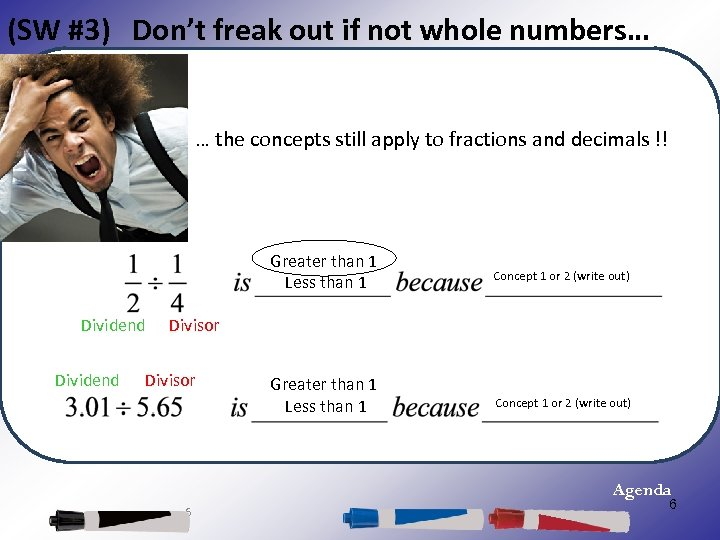(SW #3) Don’t freak out if not whole numbers… … the concepts still apply to fractions and decimals !! Greater than 1 Less than 1 Dividend Concept 1 or 2 (write out) Divisor Greater than 1 Less than 1 Concept 1 or 2 (write out) Agenda 6 6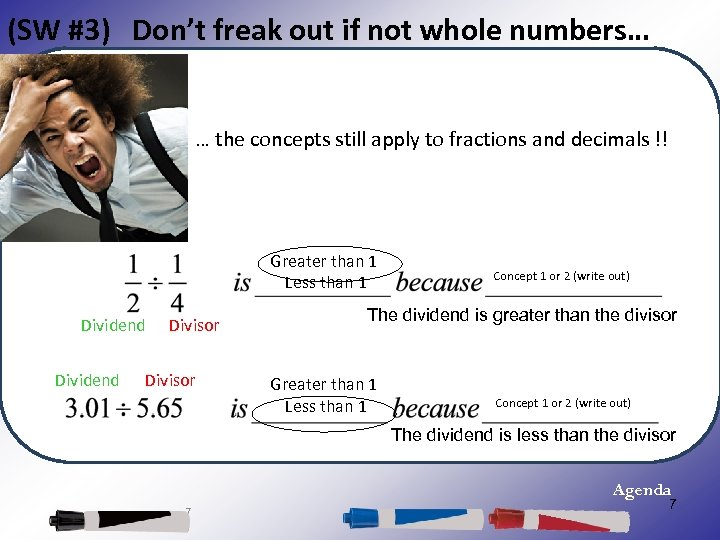(SW #3) Don’t freak out if not whole numbers… … the concepts still apply to fractions and decimals !! Greater than 1 Less than 1 Dividend Divisor Concept 1 or 2 (write out) The dividend is greater than the divisor Greater than 1 Less than 1 Concept 1 or 2 (write out) The dividend is less than the divisor Agenda 7 7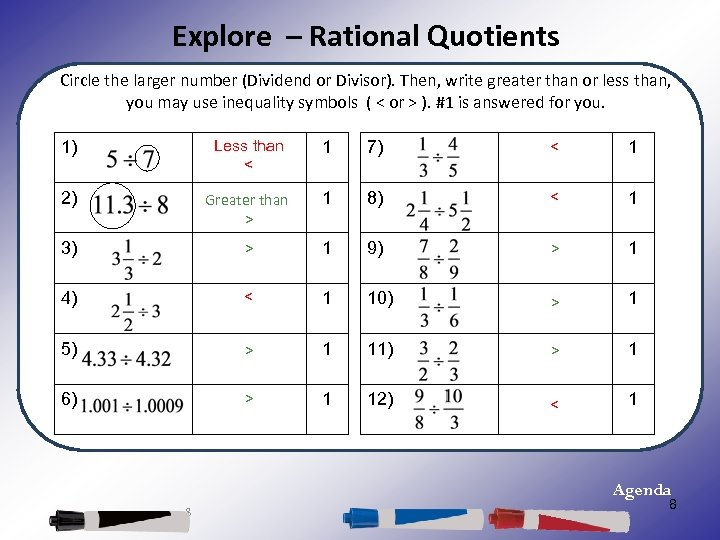Explore – Rational Quotients Circle the larger number (Dividend or Divisor). Then, write greater than or less than, you may use inequality symbols ( < or > ). #1 is answered for you. 1) Less than < 1 7) < 1 2) Greater than > 1 8) < 1 3) > 1 9) > 1 4) < 1 10) > 1 5) > 1 11) > 1 6) > 1 12) < 1 Agenda 8 8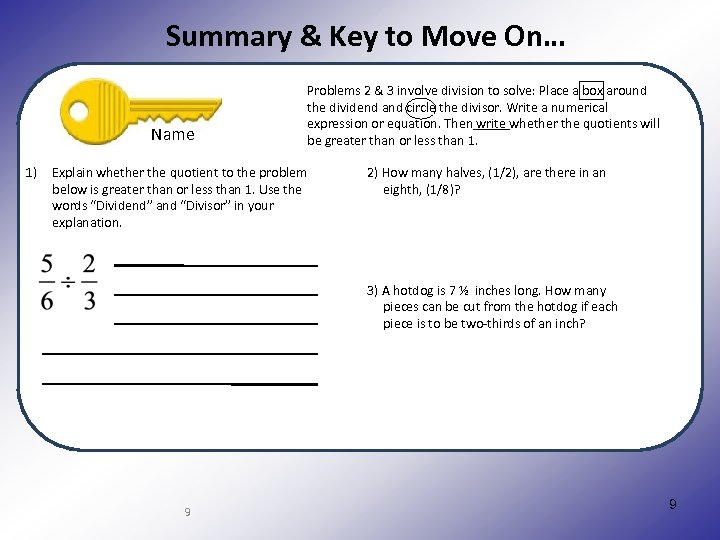Summary & Key to Move On… Name 1) Problems 2 & 3 involve division to solve: Place a box around the dividend and circle the divisor. Write a numerical expression or equation. Then write whether the quotients will be greater than or less than 1. Explain whether the quotient to the problem below is greater than or less than 1. Use the words “Dividend” and “Divisor” in your explanation. 2) How many halves, (1/2), are there in an eighth, (1/8)? An Italian sausage is 8 inches long. How many pieces of sausage can be cut from the 8 inch piece of sausage if each piece is to be 3) A hotdog is 7 ½ inches long. How many pieces can be cut from the hotdog if each piece is to be two-thirds of an inch? 9 9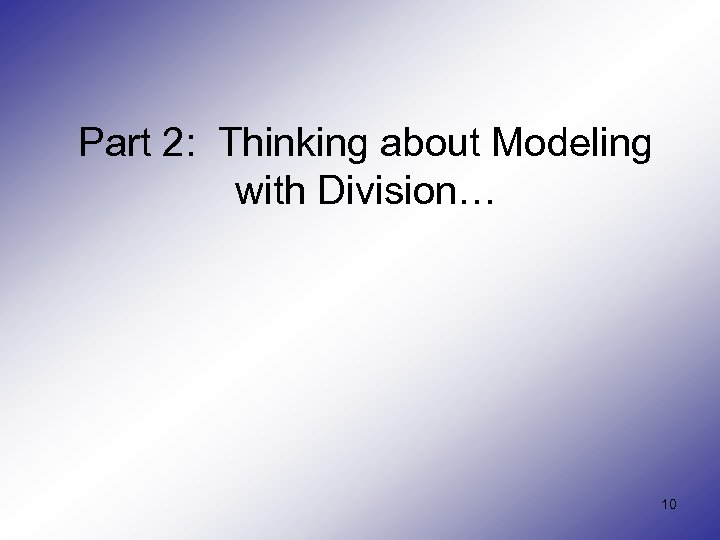Part 2: Thinking about Modeling with Division… 10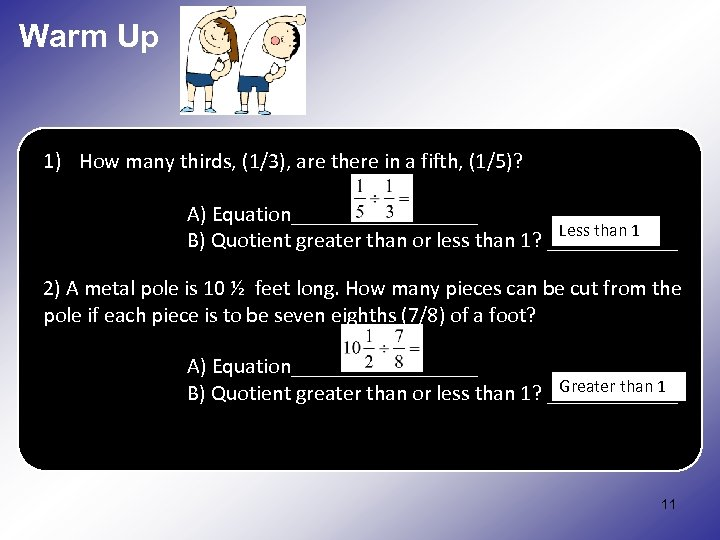Warm Up 1) How many thirds, (1/3), are there in a fifth, (1/5)? A) Equation_________ Less than 1 B) Quotient greater than or less than 1? ______ How. A metal pole is 10 ½there in an How many pieces can be cut from the 2) many halves, (1/2), are feet long. eighth, (1/ pole if each piece is to be seven eighths (7/8) of a foot? A) Equation_________ Greater than 1 B) Quotient greater than or less than 1? ______ 11 11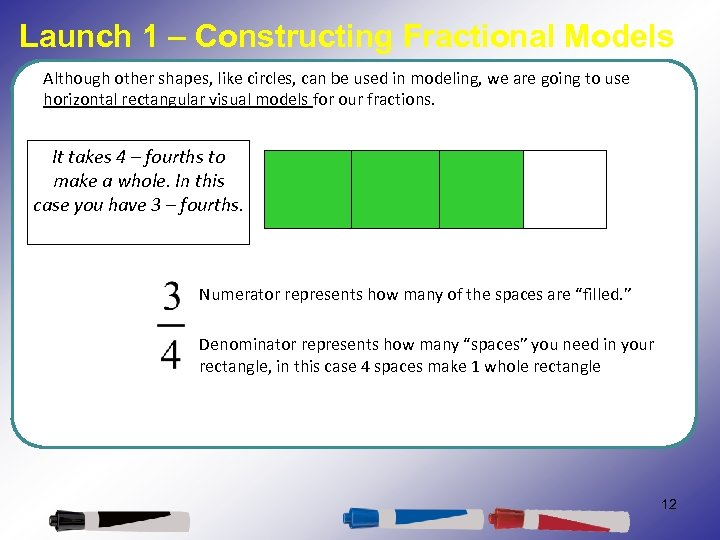Launch 1 – Constructing Fractional Models Although other shapes, like circles, can be used in modeling, we are going to use horizontal rectangular visual models for our fractions. It takes 4 – fourths to make a whole. In this case you have 3 – fourths. Numerator represents how many of the spaces are “filled. ” Denominator represents how many “spaces” you need in your rectangle, in this case 4 spaces make 1 whole rectangle 12 12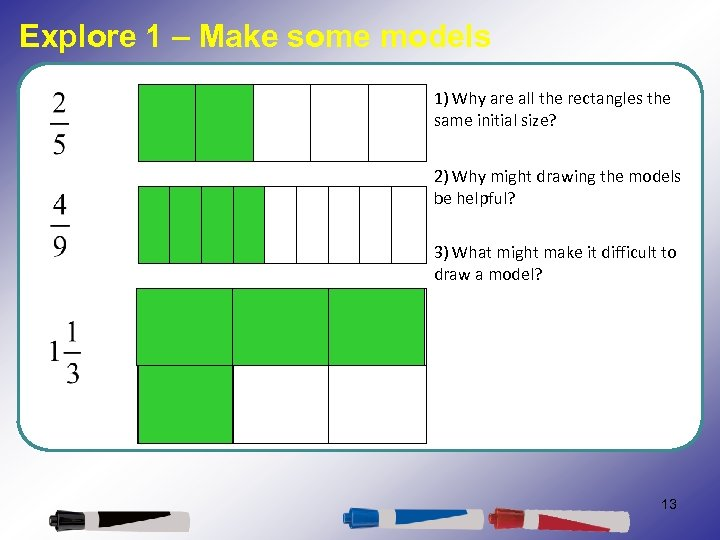Explore 1 – Make some models 1) Why are all the rectangles the same initial size? 2) Why might drawing the models be helpful? 3) What might make it difficult to draw a model? 13 13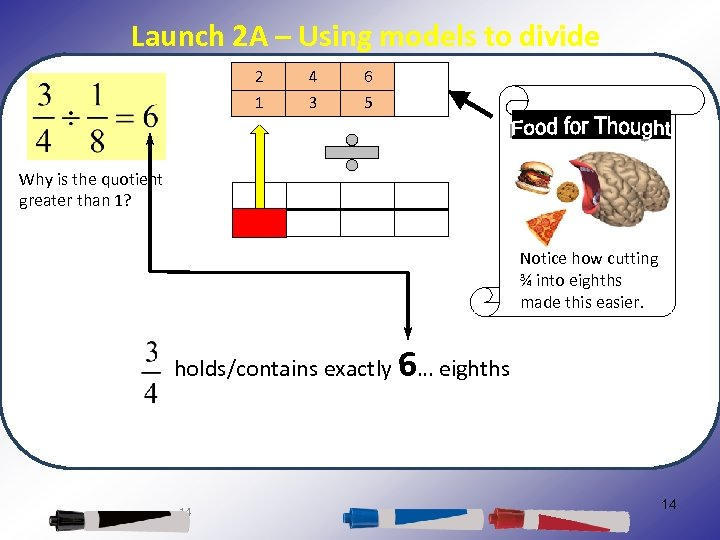Launch 2 A – Using models to divide 2 4 6 1 3 5 Why is the quotient greater than 1? Notice how cutting ¾ into eighths made this easier. holds/contains exactly 6… eighths 14 14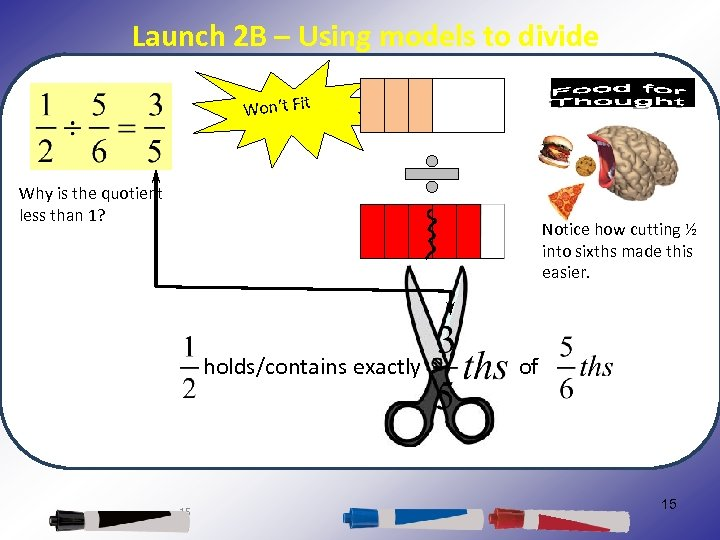Launch 2 B – Using models to divide Won’t Fit Why is the quotient less than 1? Notice how cutting ½ into sixths made this easier. holds/contains exactly 15 of 15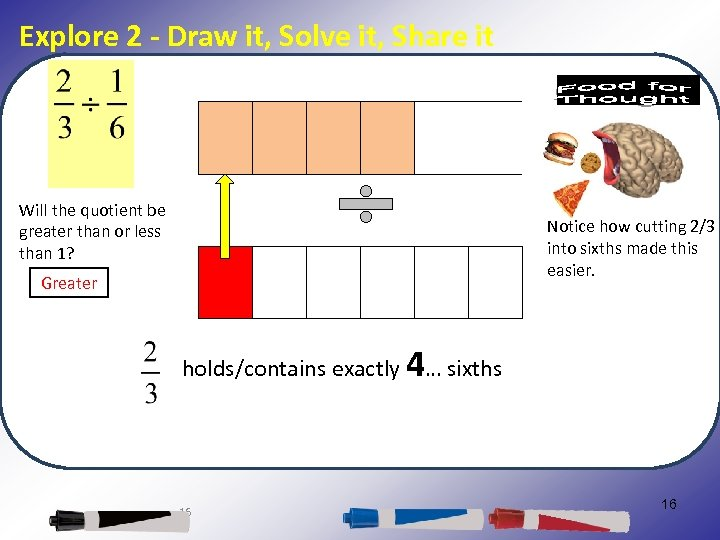Explore 2 - Draw it, Solve it, Share it Will the quotient be greater than or less than 1? Notice how cutting 2/3 into sixths made this easier. Greater holds/contains exactly 4… sixths 16 16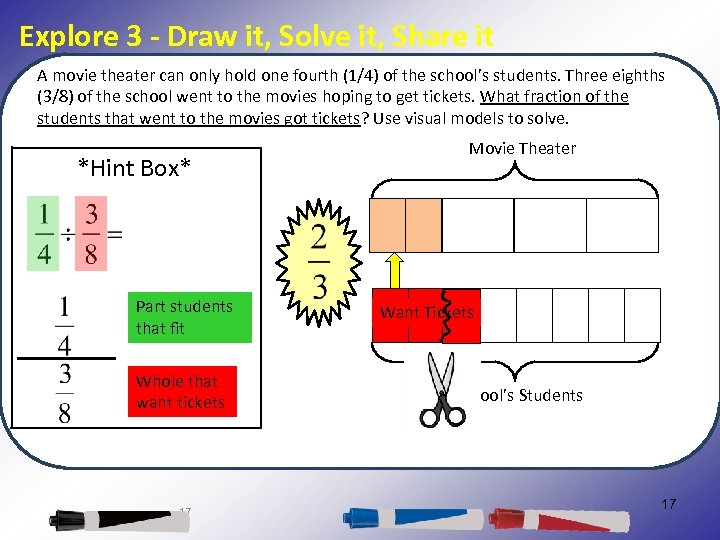Explore 3 - Draw it, Solve it, Share it A movie theater can only hold one fourth (1/4) of the school’s students. Three eighths (3/8) of the school went to the movies hoping to get tickets. What fraction of the students that went to the movies got tickets? Use visual models to solve. Movie Theater *Hint Box* Students Fit Part students that fit Whole that want tickets 17 Want Tickets School’s Students 17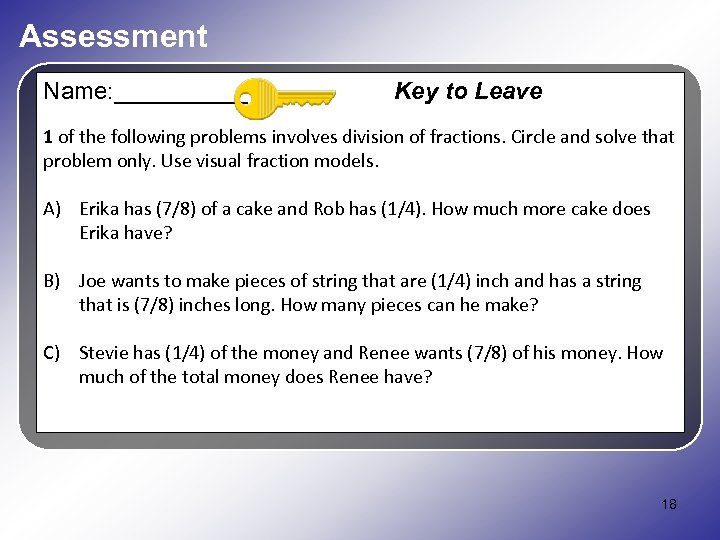Assessment Name: _____ Key to Leave 1 of the following problems involves division of fractions. Circle and solve that problem only. Use visual fraction models. A) Erika has (7/8) of a cake and Rob has (1/4). How much more cake does Erika have? B) Joe wants to make pieces of string that are (1/4) inch and has a string that is (7/8) inches long. How many pieces can he make? C) Stevie has (1/4) of the money and Renee wants (7/8) of his money. How much of the total money does Renee have? 18 18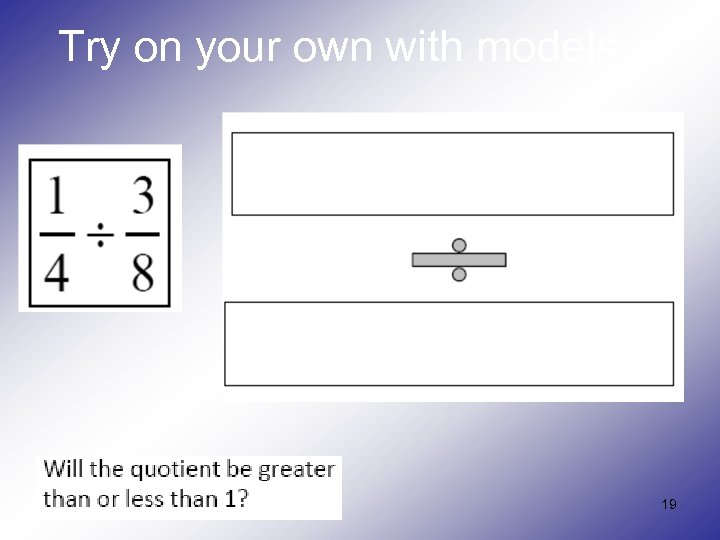Try on your own with models… 19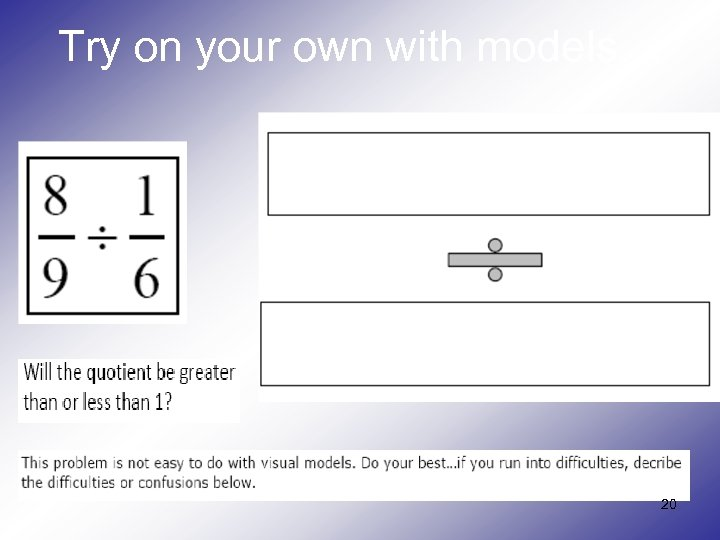Try on your own with models… 20Part 3: Thinking more about the Division of Fractions 21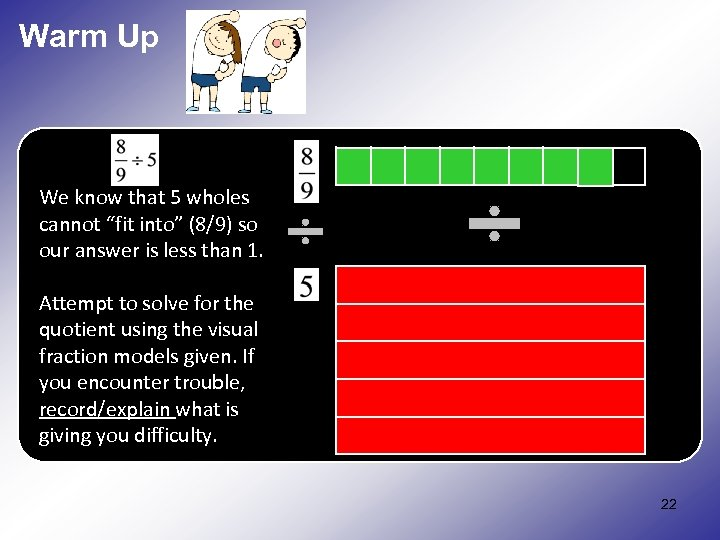Warm Up We know that 5 wholes cannot “fit into” (8/9) so our answer is less than 1. How many halves, (1/2), are there in an Attempt to solve for the eighth, (1/ quotient using the visual fraction models given. If you encounter trouble, record/explain what is giving you difficulty. 22 22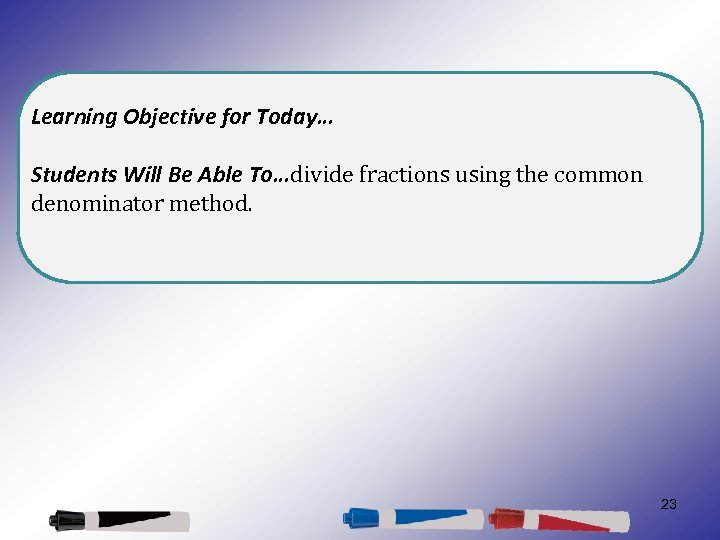Learning Objective for Today… Students Will Be Able To…divide fractions using the common denominator method. 23 23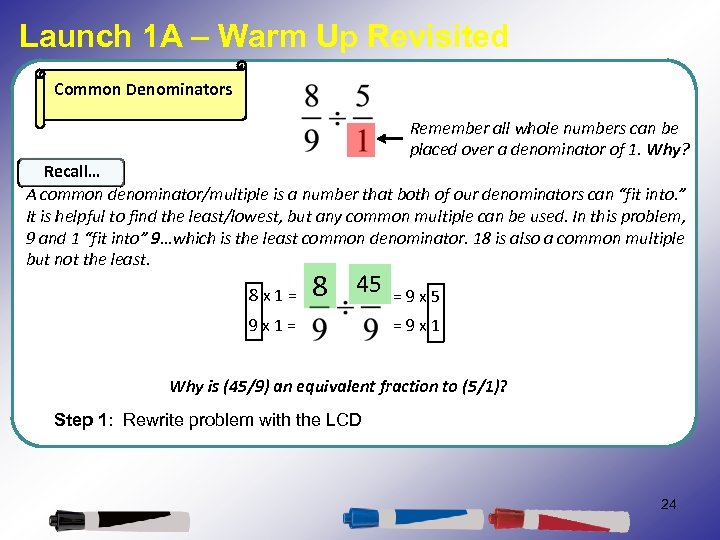Launch 1 A – Warm Up Revisited Common Denominators Remember all whole numbers can be placed over a denominator of 1. Why? Recall… A common denominator/multiple is a number that both of our denominators can “fit into. ” It is helpful to find the least/lowest, but any common multiple can be used. In this problem, 9 and 1 “fit into” 9…which is the least common denominator. 18 is also a common multiple but not the least. 8 x 1= 8 45 9 x 1= =9 x 5 =9 x 1 Why is (45/9) an equivalent fraction to (5/1)? Step 1: Rewrite problem with the LCD 24 24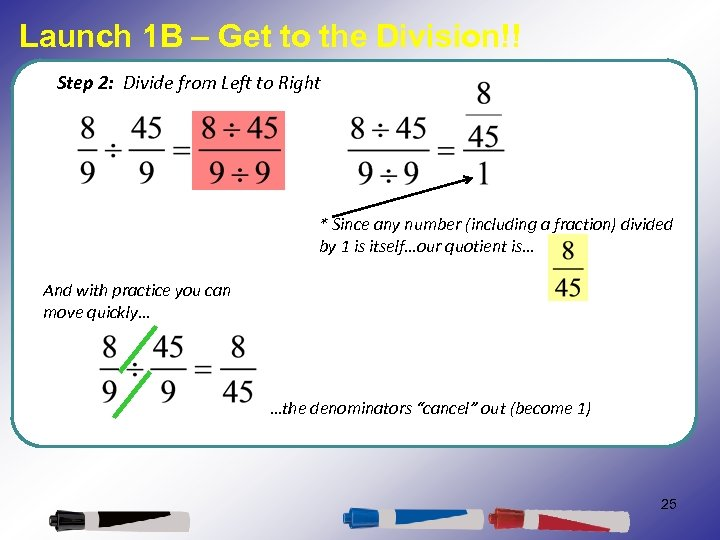Launch 1 B – Get to the Division!! Step 2: Divide from Left to Right * Since any number (including a fraction) divided by 1 is itself…our quotient is… And with practice you can move quickly… …the denominators “cancel” out (become 1) 25 25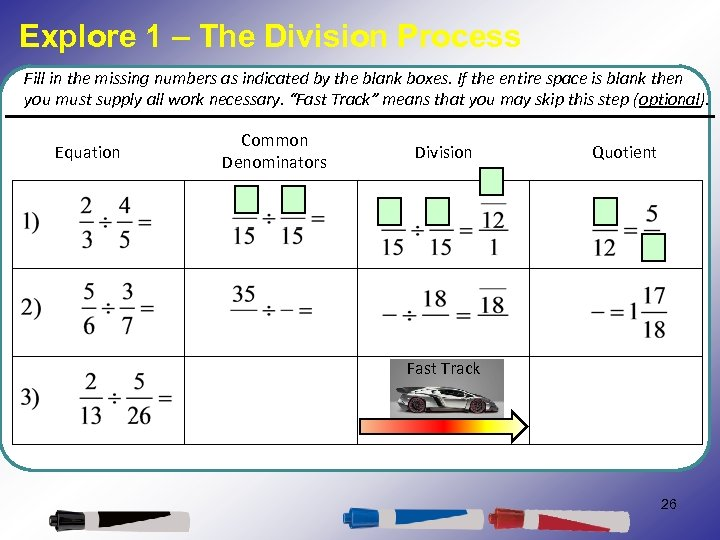Explore 1 – The Division Process Fill in the missing numbers as indicated by the blank boxes. If the entire space is blank then you must supply all work necessary. “Fast Track” means that you may skip this step (optional). Equation Common Denominators Division Quotient Fast Track 26 26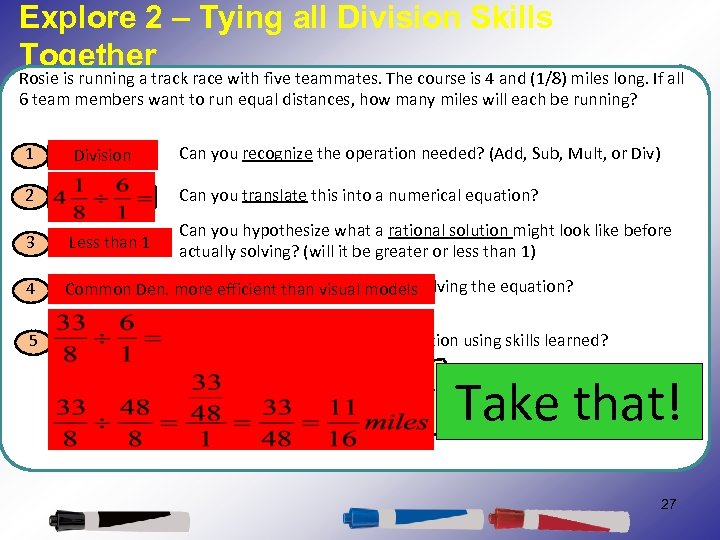Explore 2 – Tying all Division Skills Together Rosie is running a track race with five teammates. The course is 4 and (1/8) miles long. If all 6 team members want to run equal distances, how many miles will each be running? 1 Recognition Division Can you recognize the operation needed? (Add, Sub, Mult, or Div) 2 Translation Can you translate this into a numerical equation? 3 Rationality 1 Less than Can you hypothesize what a rational solution might look like before actually solving? (will it be greater or less than 1) 4 Can efficient than a method of Determine. Den. moreyou determinevisual modelssolving the equation? Common 5 Execution Can you execute the accurate solution using skills learned? Take that! If you can, prove it ! 27 27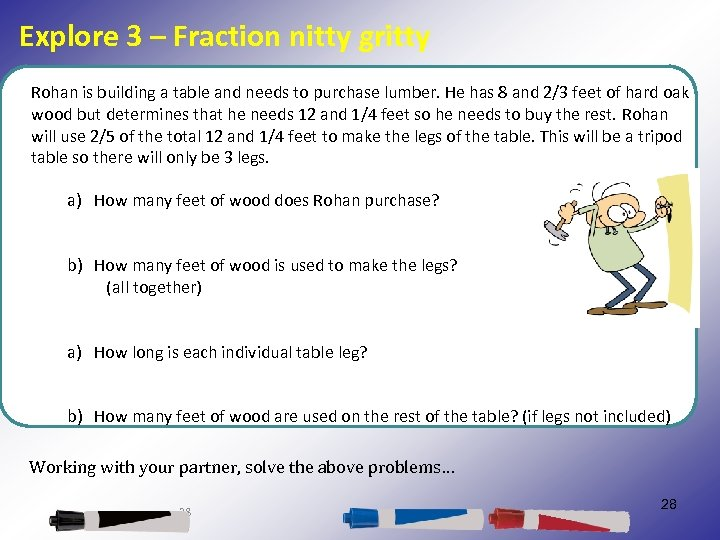Explore 3 – Fraction nitty gritty Rohan is building a table and needs to purchase lumber. He has 8 and 2/3 feet of hard oak wood but determines that he needs 12 and 1/4 feet so he needs to buy the rest. Rohan will use 2/5 of the total 12 and 1/4 feet to make the legs of the table. This will be a tripod table so there will only be 3 legs. a) How many feet of wood does Rohan purchase? b) How many feet of wood is used to make the legs? (all together) a) How long is each individual table leg? b) How many feet of wood are used on the rest of the table? (if legs not included) Working with your partner, solve the above problems… 28 28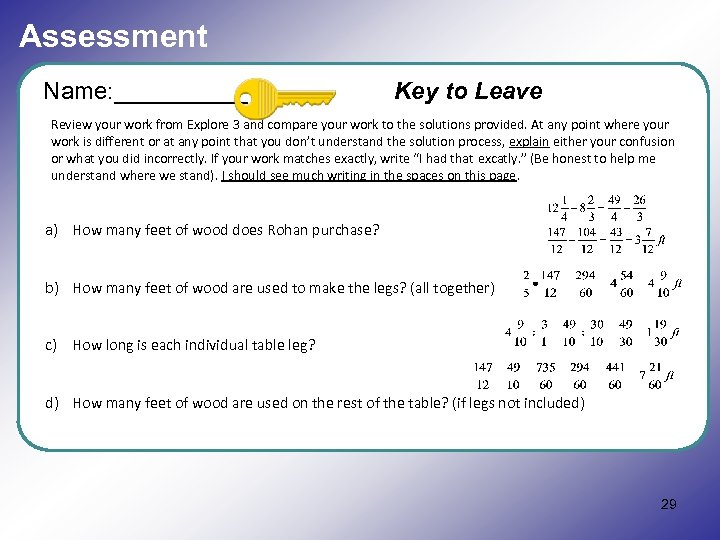Assessment Name: _____ Key to Leave Review your work from Explore 3 and compare your work to the solutions provided. At any point where your work is different or at any point that you don’t understand the solution process, explain either your confusion or what you did incorrectly. If your work matches exactly, write “I had that excatly. ” (Be honest to help me understand where we stand). I should see much writing in the spaces on this page. a) How many feet of wood does Rohan purchase? b) How many feet of wood are used to make the legs? (all together) c) How long is each individual table leg? d) How many feet of wood are used on the rest of the table? (if legs not included) 29 29